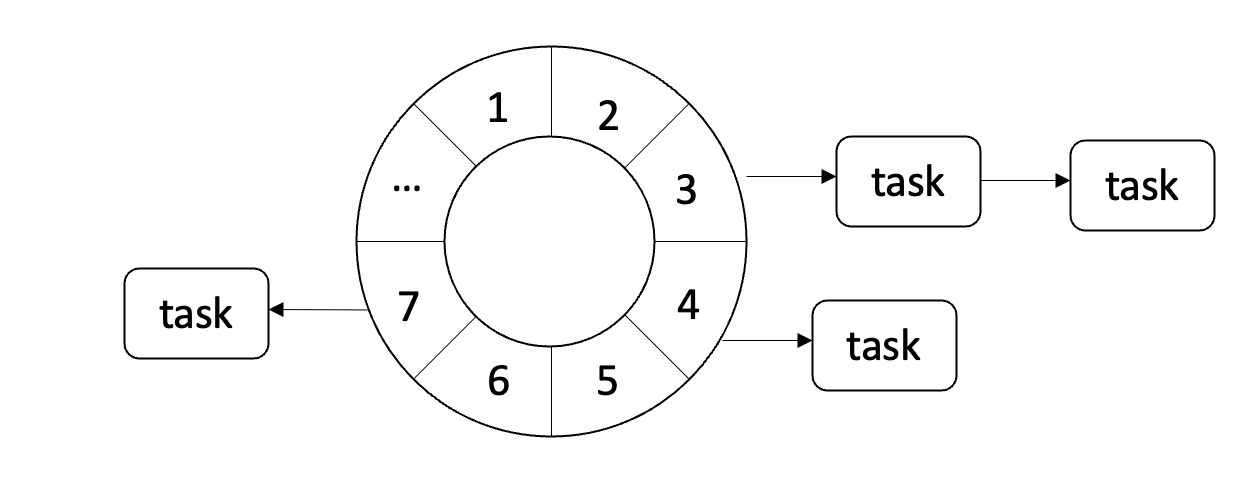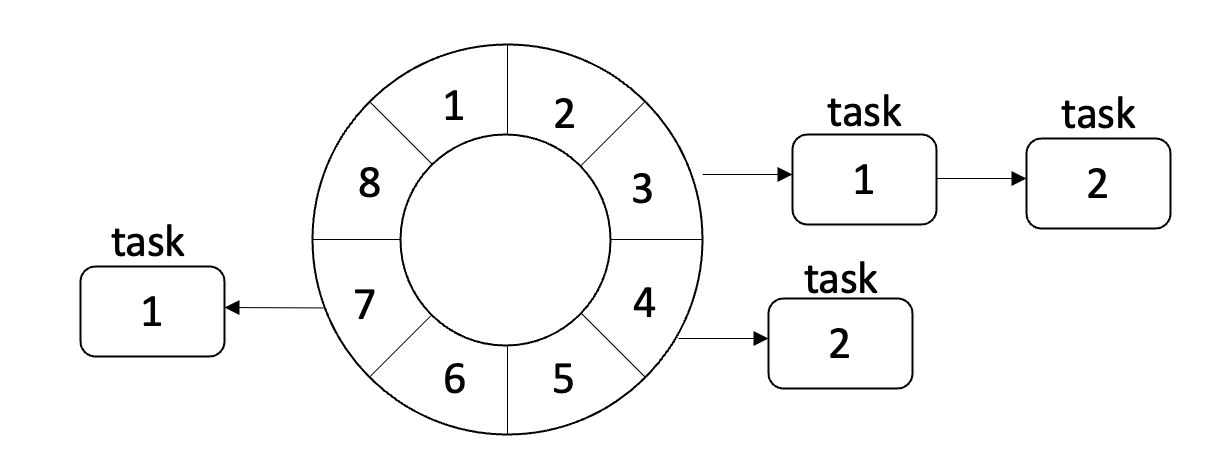# java.util.Timer

private final TaskQueue queue = new TaskQueue();


private TimerTask[] queue = new TimerTask;


    void add(TimerTask task) {
// Grow backing store if necessary
if (size + 1 == queue.length)
queue = Arrays.copyOf(queue, 2*queue.length);

fixUp(size);
}


    void removeMin() {
queue = queue[size];
queue[size--] = null;  // Drop extra reference to prevent memory leak
fixDown(1);
}


fixup的原理就是将当前的节点和它的父节点进行比较，如果小于父节点就和父节点进行交互，然后遍历进行这个过程：

    private void fixUp(int k) {
while (k > 1) {
int j = k >> 1;
if (queue[j].nextExecutionTime <= queue[k].nextExecutionTime)
break;
TimerTask tmp = queue[j];  queue[j] = queue[k]; queue[k] = tmp;
k = j;
}
}


fixDown的原理是比较当前节点和它的子节点，如果当前节点大于子节点，则将其降级：

    private void fixDown(int k) {
int j;
while ((j = k << 1) <= size && j > 0) {
if (j < size &&
queue[j].nextExecutionTime > queue[j+1].nextExecutionTime)
j++; // j indexes smallest kid
if (queue[k].nextExecutionTime <= queue[j].nextExecutionTime)
break;
TimerTask tmp = queue[j];  queue[j] = queue[k]; queue[k] = tmp;
k = j;
}
}


DelayedWorkQueue和DelayQueue,PriorityQueue一样都是一个基于堆的数据结构。

       private void siftUp(int k, RunnableScheduledFuture<?> key) {
while (k > 0) {
int parent = (k - 1) >>> 1;
RunnableScheduledFuture<?> e = queue[parent];
if (key.compareTo(e) >= 0)
break;
queue[k] = e;
setIndex(e, k);
k = parent;
}
queue[k] = key;
setIndex(key, k);
}

private void siftDown(int k, RunnableScheduledFuture<?> key) {
int half = size >>> 1;
while (k < half) {
int child = (k << 1) + 1;
RunnableScheduledFuture<?> c = queue[child];
int right = child + 1;
if (right < size && c.compareTo(queue[right]) > 0)
c = queue[child = right];
if (key.compareTo(c) <= 0)
break;
queue[k] = c;
setIndex(c, k);
k = child;
}
queue[k] = key;
setIndex(key, k);
}


# HashedWheelTimernetty提供了这种算法的实现：

public class HashedWheelTimer implements Timer


private final HashedWheelBucket[] wheel;


    private static HashedWheelBucket[] createWheel(int ticksPerWheel) {
//ticksPerWheel may not be greater than 2^30
checkInRange(ticksPerWheel, 1, 1073741824, "ticksPerWheel");

ticksPerWheel = normalizeTicksPerWheel(ticksPerWheel);
HashedWheelBucket[] wheel = new HashedWheelBucket[ticksPerWheel];
for (int i = 0; i < wheel.length; i ++) {
wheel[i] = new HashedWheelBucket();
}
return wheel;
}


HashedWheelBucket中的每个元素都是一个HashedWheelTimeout. HashedWheelTimeout中有一个remainingRounds属性用来记录这个Timeout元素还会在Bucket中保存多久。

long remainingRounds;


# 总结

netty中的HashedWheelTimer可以实现更高效的Timer功能，大家用起来吧。